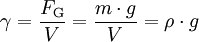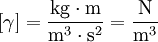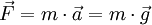# What is mass weight

The Weight γ, also specific weight, is the ratio of the weight of a body to its volume.

### Basics and definition

In contrast to the density ρ, which is the mass m based on volume V is (unit: kg / m³), ​​is the Weights the weight F.G based on volume V (Unit: N / m³, formerly kp / m³), ​​that is, density and specific gravity differ in the value of the gravitational acceleration Gwhich influences the size of the weights.The symbol of the weight is γ (Greek. gamma)

The unit of the weights results as follows:### Weight in distinction to density

The Weights In contrast to the density, it is location-dependent, since the acceleration due to gravity is not the same everywhere. On earth, the weight would be based on a mass of 1 kg according to the formula “force is mass m times acceleration awhere on the earth's surface the acceleration due to gravity is the acceleration due to gravity G = 9.81 m / s² must be used:

F. = 1 kg · 9.81 m / s² = 9.81 kg m / s² = 9.81 N (outdated:… = 1 kp = 9.81 N)

On earth, a mass of 1 kilogram has "the weight" 1 kp = 9.81 Newtons, ie about 10 Newtons.

A little less colloquial and expressed a little more physically, one would say:

• A mass of 1 kg goes in the area of ​​gravitational acceleration G a weight of 9.81 N.

If this mass of 1 kg is brought to the moon (lunar gravitational acceleration, "lunar gravitational force" = 1/6 G), then assume only 9.81 N / 6 = 1.635 N weight force, so the mass is consequently lighter, although its dimensions have not changed. It has become (only) hers Weights changed.

The same applies to the way to the moon: on the way to the moon, the mass is outside the gravitational pull of the moon and outside the gravitational pull of the earth, i.e. in weightless space. As a result, it no longer has any weights, better, its weight is zero. Now it no longer exerts any weight (colloquial: it “no longer has any weight”), although its density - and thus also its dimensions - have remained the same.

### Dependence of weight and weight on other sizes

In fact, the above explanation is a simplification: on earth, the displaced air exerts a corresponding lift on the mass. Therefore the weight force actually caused by the mass (its “weight”) is lower by the amount of buoyancy. The specific weight is therefore given in relation to normal air pressure (at sea level) and normal temperature 0 ° C or room temperature 21 ° C or standard temperature 25 ° C or 20 ° C.

In addition, the specific gravity is also temperature-dependent, since the volume of a body changes with temperature. If the original specific gravity has changed due to an increase in the dimensions, e.g. due to an increase in temperature, a relative density can also be derived from this.